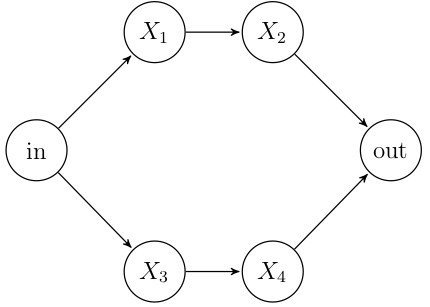1. Suppose an exam has 6 multiple-choice questions, and that each question has 5 possible answers. On each question, only 1 answer is correct. If a student guesses randomly and independently from question to question,find the probability of

1. Being correct only on questions 1 and 4.
2. Being correct on any two questions.
2. It is claimed that 80% of American drivers engage in multitasking, driving while eg talking on their cell phone, eating a snack, or texting. In a random sample of 20 drivers, let $$X$$ equal the number of multitaskers.

1. What type of random variable is $$X$$?
2. Calculate the probability that
• $$P(X = 15), \text{ eg } f(15)$$
• $$P(X > 15)$$
• $$P(X \leq 15)$$
3. Give values for the expected value, variance, and standard deviation.
3. Assume the random variables $$X_n$$ are independent, for $$n = 1, 2, 3, 4$$, and that $$P(X_n = 1) = p_n$$, where $$1$$ indicates success. Calculate the probability of success of the entire system.4. Assume $$X \sim \text{Bernoulli}(p)$$.
1. Calculate the variance of a Bernoulli(p) random variable.
2. Plot using bp.curve() the variance as a function of $$p$$, across the domain $$[0, 1]$$.
3. Guess the value of $$p$$ for which the variance is maximized.
4. Use calculus to find the value of $$p$$ for which the variance is maximized.